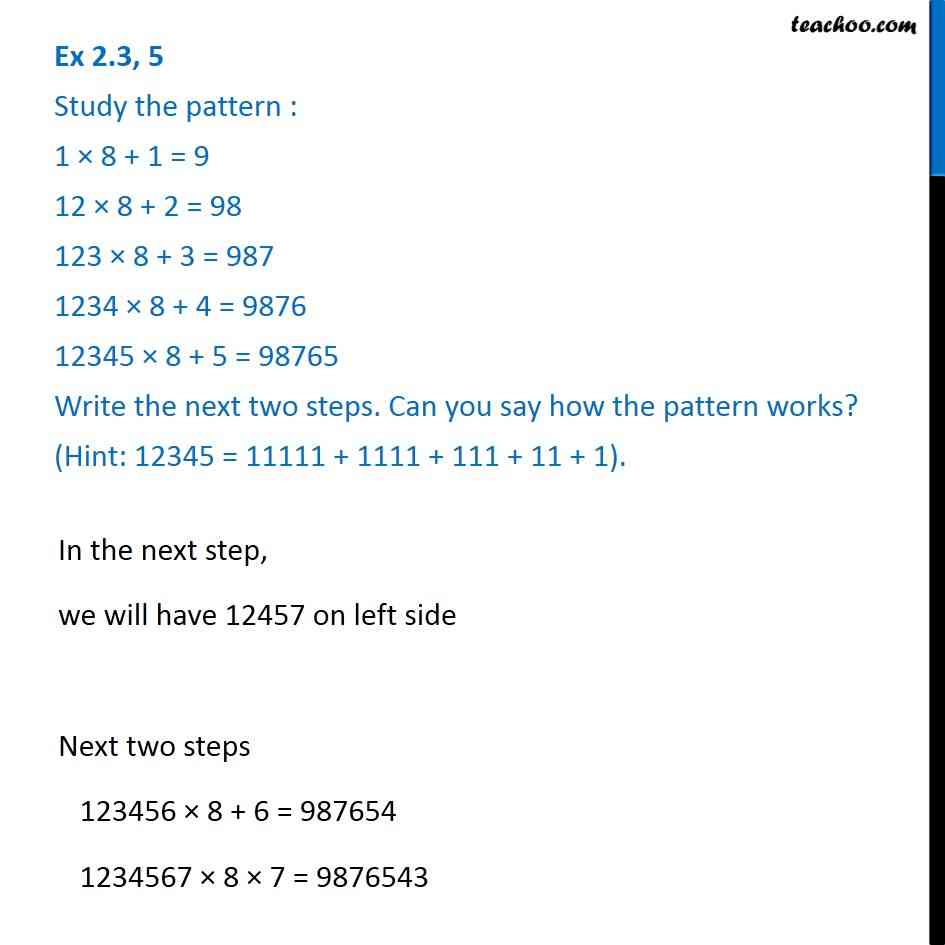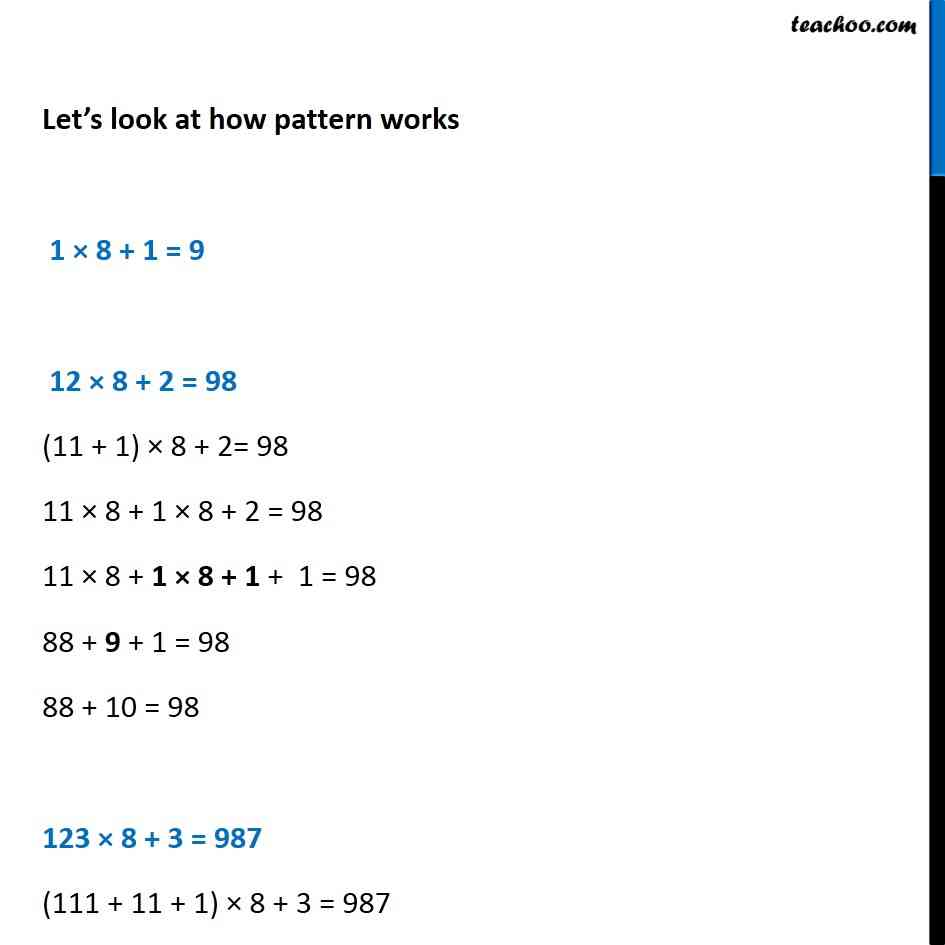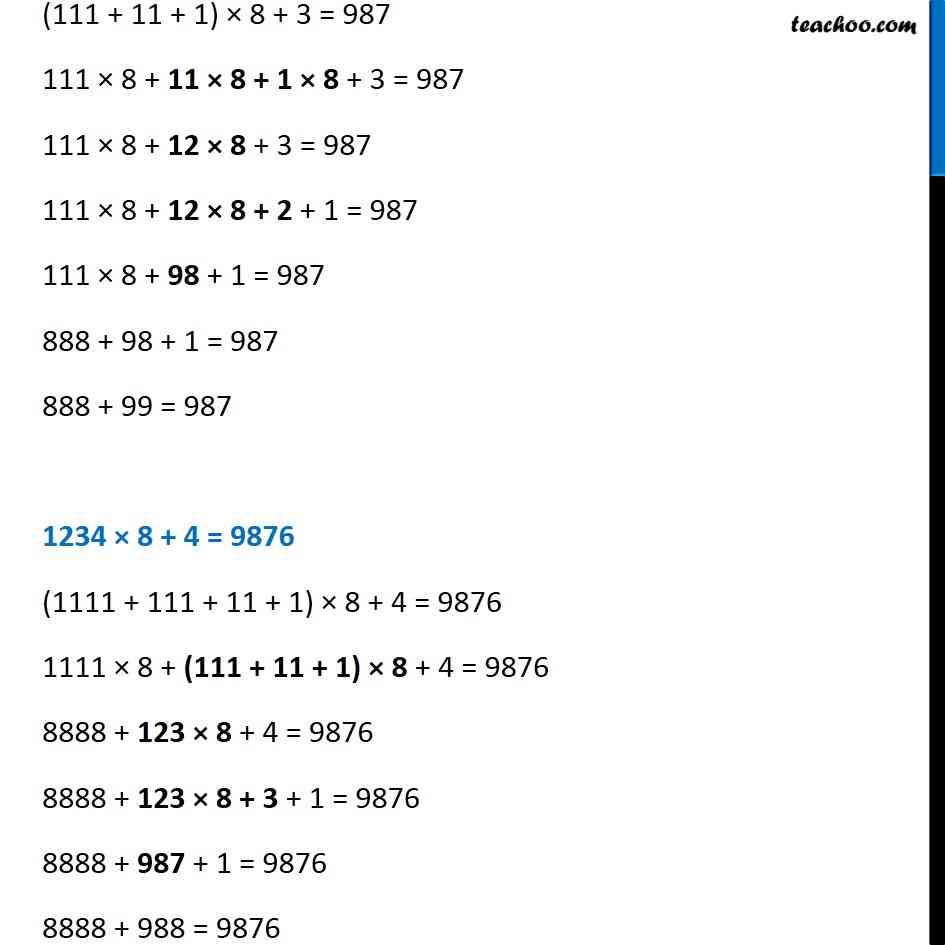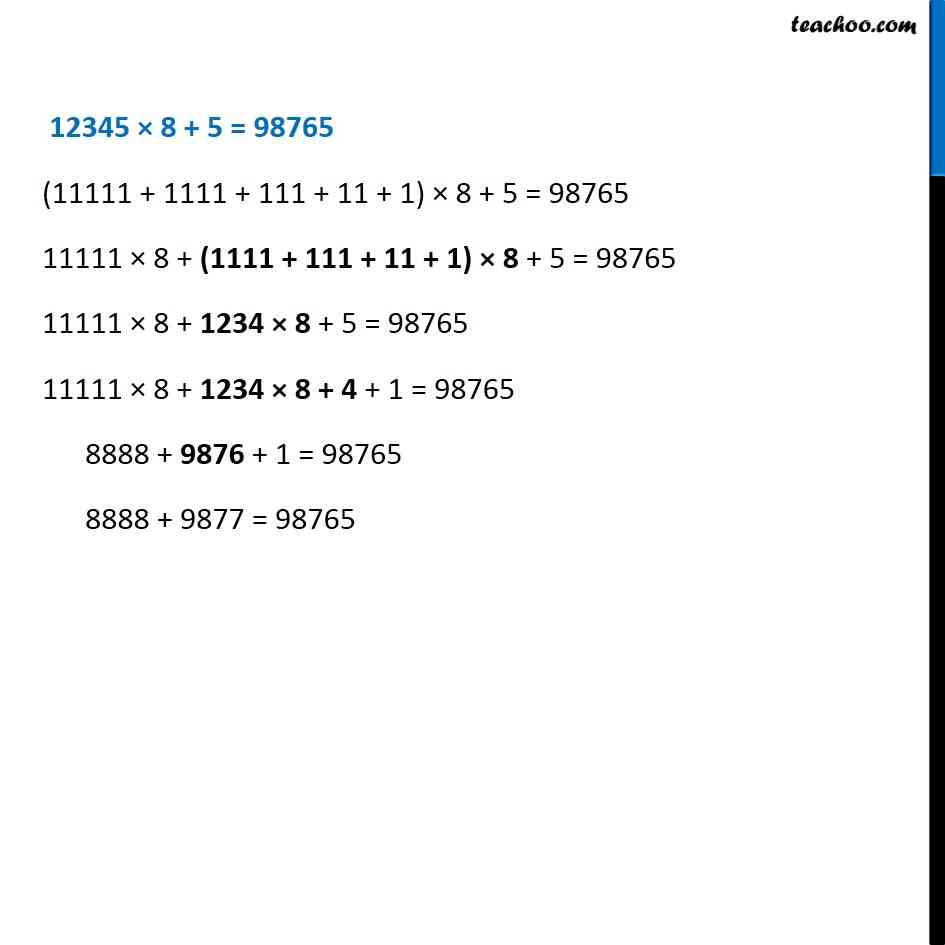1. Chapter 2 Class 6 Whole Numbers
2. Serial order wise
3. Ex 2.3

Transcript

Ex 2.3, 5 Study the pattern : 1 × 8 + 1 = 9 12 × 8 + 2 = 98 123 × 8 + 3 = 987 1234 × 8 + 4 = 9876 12345 × 8 + 5 = 98765 Write the next two steps. Can you say how the pattern works? (Hint: 12345 = 11111 + 1111 + 111 + 11 + 1). Let’s look at how pattern works 1 × 8 + 1 = 9 12 × 8 + 2 = 98 (11 + 1) × 8 + 2= 98 11 × 8 + 1 × 8 + 2 = 98 11 × 8 + 1 × 8 + 1 + 1 = 98 88 + 9 + 1 = 98 88 + 10 = 98 123 × 8 + 3 = 987 (111 + 11 + 1) × 8 + 3 = 987 (111 + 11 + 1) × 8 + 3 = 987 111 × 8 + 11 × 8 + 1 × 8 + 3 = 987 111 × 8 + 12 × 8 + 3 = 987 111 × 8 + 12 × 8 + 2 + 1 = 987 111 × 8 + 98 + 1 = 987 888 + 98 + 1 = 987 888 + 99 = 987 1234 × 8 + 4 = 9876 (1111 + 111 + 11 + 1) × 8 + 4 = 9876 1111 × 8 + (111 + 11 + 1) × 8 + 4 = 9876 8888 + 123 × 8 + 4 = 9876 8888 + 123 × 8 + 3 + 1 = 9876 8888 + 987 + 1 = 9876 8888 + 988 = 9876

Ex 2.3